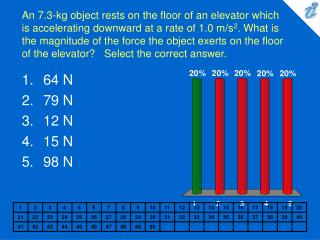DownloadDownload Presentation64 N 79 N 12 N 15 N 98 N

64 N 79 N 12 N 15 N 98 N

Télécharger la présentation64 N 79 N 12 N 15 N 98 N

- - - - - - - - - - - - - - - - - - - - - - - - - - - E N D - - - - - - - - - - - - - - - - - - - - - - - - - - -
Presentation Transcript

1. An 7.3-kg object rests on the floor of an elevator which is accelerating downward at a rate of 1.0 m/s2. What is the magnitude of the force the object exerts on the floor of the elevator? Select the correct answer. • 64 N • 79 N • 12 N • 15 N • 98 N

2. In the figure, the coefficient of kinetic friction between the surface and the larger block is 0.18, and the coefficient of kinetic friction between the surface and the smaller block is 0.31. If F = 19 N and M = 1.3 kg, what is the magnitude of the acceleration of either block? Select the correct answer. • 1.6 m/s2 • 3.4 m/s2 • 2.5 m/s2 • 1.8 m/s2 • 2.0 m/s2

3. Two bodies, A and B , collide as shown in the figures (a) and (b) below. Which statement is true? Select the correct answer. • They exert an equal and opposite force on each other in both (a) and (b). • They exert an equal and opposite forces on each other in (a) but not in (b). • They exert an equal and opposite force on each other in (b) but not in (a). • The forces are equal and opposite to each other in (a), but only the components of the forces parallel to the velocities are equal in (b). • The forces are equal and opposite in (a), but only the components of the forces perpendicular to the velocities are equal in (b).

4. The apparent weight of a fish in an elevator is greatest when the elevator _____. Select the correct answer. • moves upward at constant velocity • moves downward at constant velocity • accelerates upward • accelerates downward • is not moving

5. A box rests on the (horizontal) back of a truck. The coefficient of static friction between the box and the surface on which it rests is 0.31. What maximum distance can the truck travel (starting from rest and moving horizontally with constant acceleration) in 2.8 s without having the box slide? Select the correct answer. • 12 m • 24 m • 11 m • 33 m • 15 m

6. The horizontal surface on which the objects slide is frictionless. If M = 1.4 kg and the magnitude of the force of the small block on the large block is 4.7 N, determine F. Select the correct answer. • 7.1 N • 1.6 N • 3.3 N • 7.7 N • 3.1 N

7. A 2.9-kg object has a velocity of 3.3j m/s at t = 0. A constant resultant force of (2.3 i + 4.6 j ) N then acts on the object for 3.6 s. What is the magnitude of the object's velocity at the end of the 3.6-s interval? Select the correct answer. • 9.5 m/s • 3.7 m/s • 5.0 m/s • 8.6 m/s • 5.7 m/s

8. The tension in a string from which a 4.4-kg object is suspended in an elevator is equal to 53 N. What is the acceleration of the elevator? Select the correct answer. • 2.2 m/s2 upward • 12 m/s2 upward • 2.0 m/s2 downward • 3.8 m/s2 upward • 3.6 m/s2 downward

9. If α= 40°, β = 63° and M = 4.3 kg, determine the tension in string 1. Select the correct answer. • 20 N • 17 N • 41 N • 21 N • 39 N

10. If α= 44° and the tension in string 2 is 25 N, determine M. Select the correct answer. • 2.5 kg • 3.7 kg • 2.1 kg • 3.5 kg • 2.6 kg

11. A 2.3-kg block is projected up a rough 14° inclined plane. As the block slides up the incline, its acceleration is 4.8 m/s2 down the incline. What is the magnitude of the force of friction acting on the block? Select the correct answer. • 5.6 N • 2.7 N • 5.5 N • 2.9 N • 8.1 N